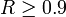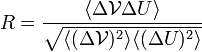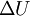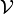# Strongly correlating liquids

(diff) ← Older revision | Latest revision (diff) | Newer revision → (diff)
Strongly correlating liquids are defined as those for whom$R \ge 0.9$ where (Eq. 6 )$R=\frac{\langle \Delta \mathcal{V} \Delta U \rangle}{ \sqrt{ \langle (\Delta \mathcal{V})^2 \rangle \langle (\Delta U)^2 \rangle } }$
where$\Delta U$ represents fluctuations of the potential in the NVT ensemble and$\mathcal{V}$ is the virial (see also Virial pressure).